# Basic Multiplication Math Drill Worksheets

The multiplication math facts worksheets created in this section help students master the instant recall of their multiplication facts up to 12 x 12.

## Multiplication facts review tables

The tables on this page summarize multiplication facts in various ranges, 0-7, 1-10, and 1-12 with and without answers. This table shows multiplication facts 0-7 with answers: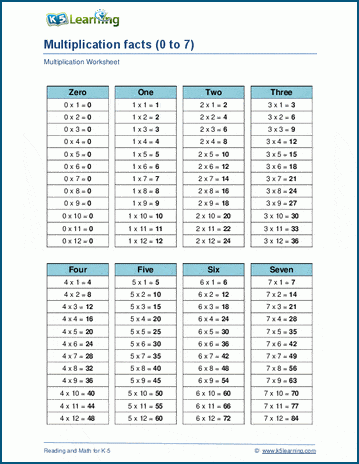## Multiplication times tables in order

We have created two separate pages of worksheets covering multiplication in table format of 1-10 and 1-12. Each worksheets contains full, 50%, 33%, 10% hints and no hints. Here’s what the full table of 1-10 multiplication looks like: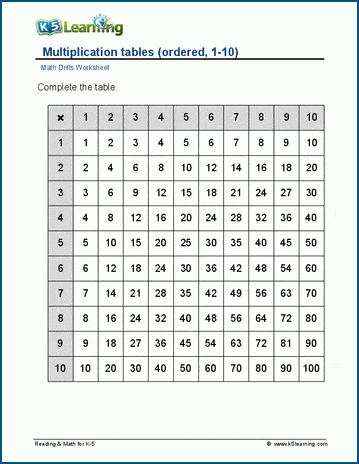## Multiplication times tables in random order

This section contains four pages of random order tables: multiplication of 1-10 with hints, multiplication of 1-10 without hints, multiplication of 1-12 with hints, and multiplication of 1-12 without hints. This random multiplication table of 1-12 contain 50% hints.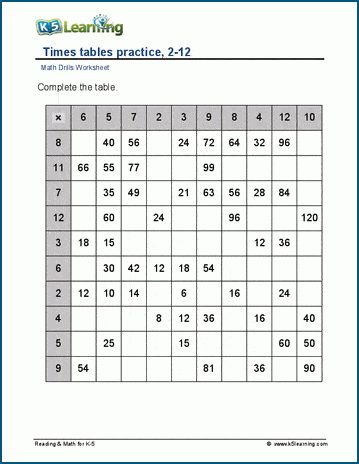## Multiplication facts practice worksheets on the vertical

Now we move onto multiplying on the vertical, starting with multiplication 0-5, then 0-7, then 1-10 and finally 2-12 worksheets. Each page contains worksheets of 49 and 100 questions. This is one of the multiplication facts on the vertical worksheet with 49 questions: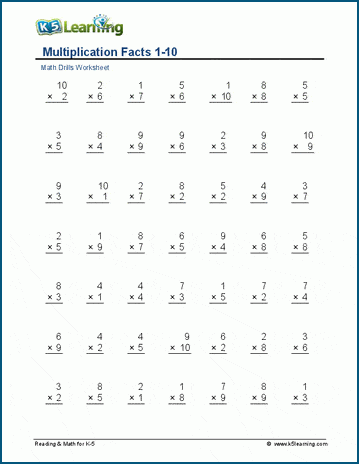## Multiplication facts practice worksheets on the horizontal

Similar to the above worksheets, these ones have students working on multiplication math facts on the horizontal, starting with 0-5, then 0-7, then 1-10 and finally 1-12. We have created separate worksheets for practicing with zeros and without. This is what one of the 0-7 multiplication worksheets that include zeros looks like: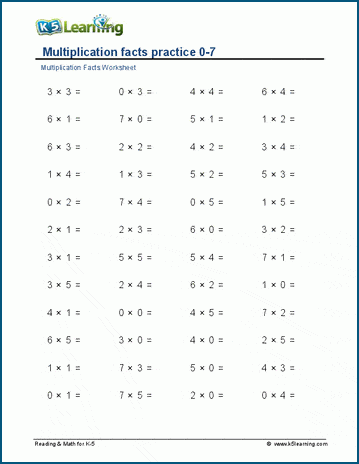## Practice multiplication by single numbers

The next section of worksheets has students practice individual numbers. For example, this page of 6 times tables and worksheets. This is the 6 times table worksheet.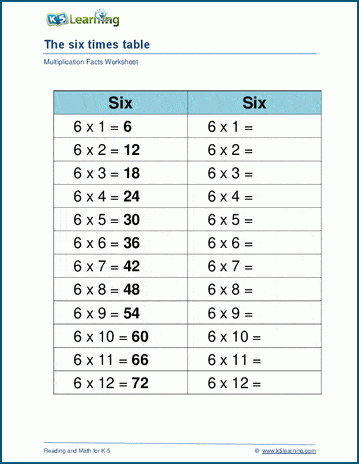## Worksheets focusing on pairs of numbers in multiplication

This section works on pairs of numbers in multiplication worksheets, such as these multiply by 4 and 6 worksheets: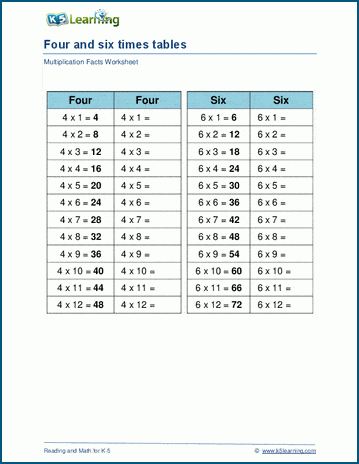## Worksheets focusing on multiplying groups of numbers

This section practices 3 multiples at a time, such as these multiply by 7, 8, and 9 worksheets: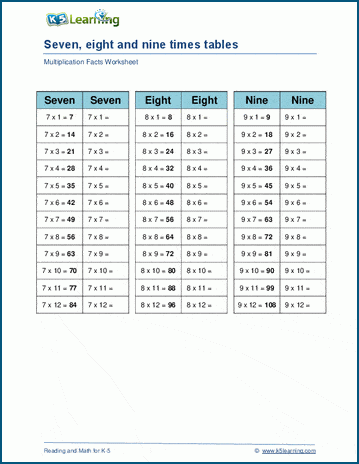## Multiplication circle drills

Circle drills are a great way to practice math facts. In this section we have two separate pages of 1-10 and 2-12 multiplication circle drill worksheets. Each page contains different difficult levels. This is one of the 1-10 circle drill worksheets: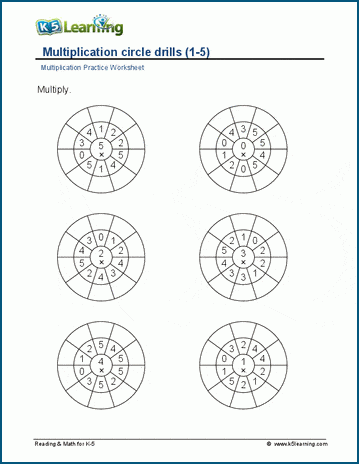## Practice missing factor questions in multiplication questions

The final section contains worksheets at increasing difficulty levels of missing factor questions. This worksheet has students practice missing factors from 1-12: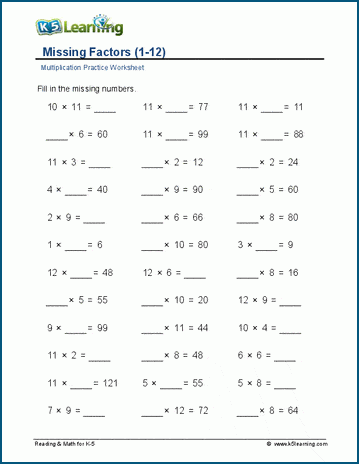Become a Member

This content is available to members only.